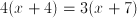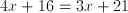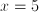Example Questions

Example Question #1 : Binomials

Solve for.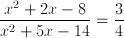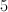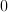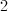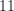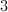Explanation:Factor the expression

numerator: find two numbers that add to 2 and multiply to -8 [use 4,-2]

denominator: find two numbers that add to 5 and multiply to -14 [use 7,-2]

new expression: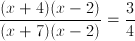Cancel the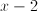and cross multiply.